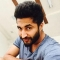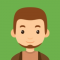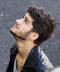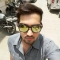## Smith Numbers in javaUpdated on 25-Jun-2020 12:19:59

807 Views

A composite number whose sum of digits equal to the sum of the digits of its prime factors.Ex: 58 = 2 x 29 (5 + 8 = 12) (2+ 2 + 9 = 12)Programpublic class SmithNumbers {    public static boolean isPrime(int number) {       int loop;       int prime = 1;       for(loop = 2; loop < number; loop++) {          if((number % loop) == 0) {             prime = 0;          }       }       if (prime ... Read More

## Prime factors in javaUpdated on 25-Jun-2020 12:13:57

17K+ Views

Factors are the numbers we multiply to get another number.factors of 14 are 2 and 7, because 2 × 7 = 14.Some numbers can be factored in more than one way.16 can be factored as 1 × 16, 2 × 8, or 4 × 4.A number that can only be factored as 1 times itself is called a prime number.The first few primes are 2, 3, 5, 7, 11, and 13.The list of all the prime-number factors of a given number is the prime factors of a number. The factorization of a number into its prime factors and expression of ... Read More

## Deleting a File in javaUpdated on 25-Jun-2020 12:12:22

73 Views

You can delete a file using the delete() method of the File class.ProgramLive Demoimport java.io.File; public class DeleteFileExample {    public static void main(String[] args) {       try {          File file = new File("myFile");          if(file.delete()) {             System.out.println(file.getName() + " is deleted!");          } else {             System.out.println("Delete operation is failed.");          }          catch(Exception e) {             e.printStackTrace();       }    } }   OutputDelete operation is failed.

## Copying a File in javaUpdated on 25-Jun-2020 12:11:19

112 Views

Following Java program copies a file to another.Programimport java.io.File; import java.io.FileInputStream; import java.io.FileOutputStream; import java.io.IOException; public class CopyExample {    public static void main(String[] args) {       FileInputStream ins = null;       FileOutputStream outs = null;       try {          File infile = new File("C:\Users\TutorialsPoint7\Desktop\abc.txt");          File outfile = new File("C:\Users\TutorialsPoint7\Desktop\bbc.txt");          ins = new FileInputStream(infile);          outs = new FileOutputStream(outfile);          byte[] buffer = new byte;          int length;           ... Read More

## Reading a Text file in javaUpdated on 25-Jun-2020 12:09:36

988 Views